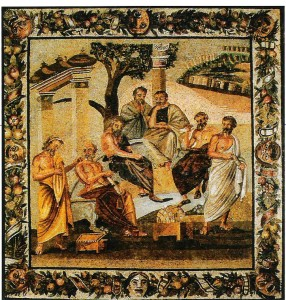#### Math Teaching Helps:

Here are Oliver’s reasons to study math >>.

Dr. Henke shares a list of recommended resources* for advanced study that he freely shared with his students and their parents:

*Affiliate links: While your price remains the same, any purchases made on Amazon.com that originate from these links result in tjed.org receiving a portion of the proceeds. So if you’re planning to shop Amazon, please start here! Thanks for supporting TJEd.

• Schneider, A Beginner’s Guide to Constructing the Universe
•Dunham, The Mathematical Universe
• Benjamin, Secrets of Mental Math
• Penrose, The Road to Reality
• Plato, Timaeus; First mathematical treatise of western thought
• Aristotle, Analytica Priora et Posteriora; System of deductive logic and method
• Euclid, The Elements (in three volumes); Exhaustive compliation of Greek geometry
• Archimedes, The Sand Reckoner; Introduces base-ten system, principle of the logarithm
• Archimedes, Method; Foundation of integral calculus
• Apollonius, Conic Sections; Exhaustive work on the conic sections
• Nicomachus, Introduction to Arithmetic; Compilation of arithmetic principles
• Ptolemy, The Almagest; Greek trigonometry used to record star positions
• al-Khowarizmi, al-jabr w’al-muqabalah; First treatise on algebra and Hindu-Arabic numerals
• Fibonacci, Libro di bacci; Introduces Hindu-Arabic numerals to Europe
• da Vinci, Linear Perspective; The mathematical elements of painting
• Stevin, La Disme; Systematic treatment of decimal fractions
• Cardano, Ars Magna; Solves cubic and bi-quadratic equations, imaginary numbers
• Galileo, Two New Sciences; Challenges Aristotle’s law of falling bodies
• Descartes, Geometry; Lays the foundation of analytical geometry
• Fermat, On the Theory of Probabilities; Pascal lays the foundation of probability
• Newton, The Principia; Invention of calculus and defines gravity
• Bernoulli, Law of Large Numbers; Lays the foundation of statistics
• Euler, Seven Bridges of Konigsberg; Lays the foundation of topology
• La Place, Celestial Mechanics; Applies calculus to heavenly orbits
• La Place (Sommerville translation), Celestial Mechanics [click here for online text]; Expansion of La Place’s work
• Boole, Symbolic Logic; Discovery of symbolic and boolean logic
• Abbott, Flatland; Fictional work about mathematical dimensions